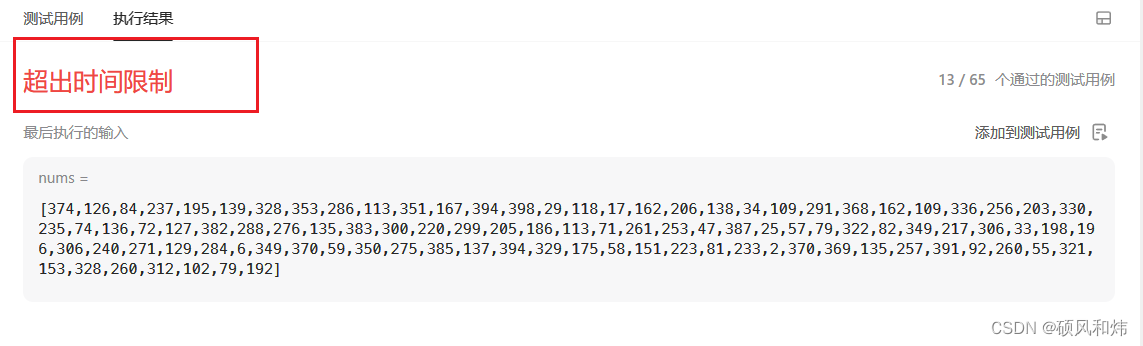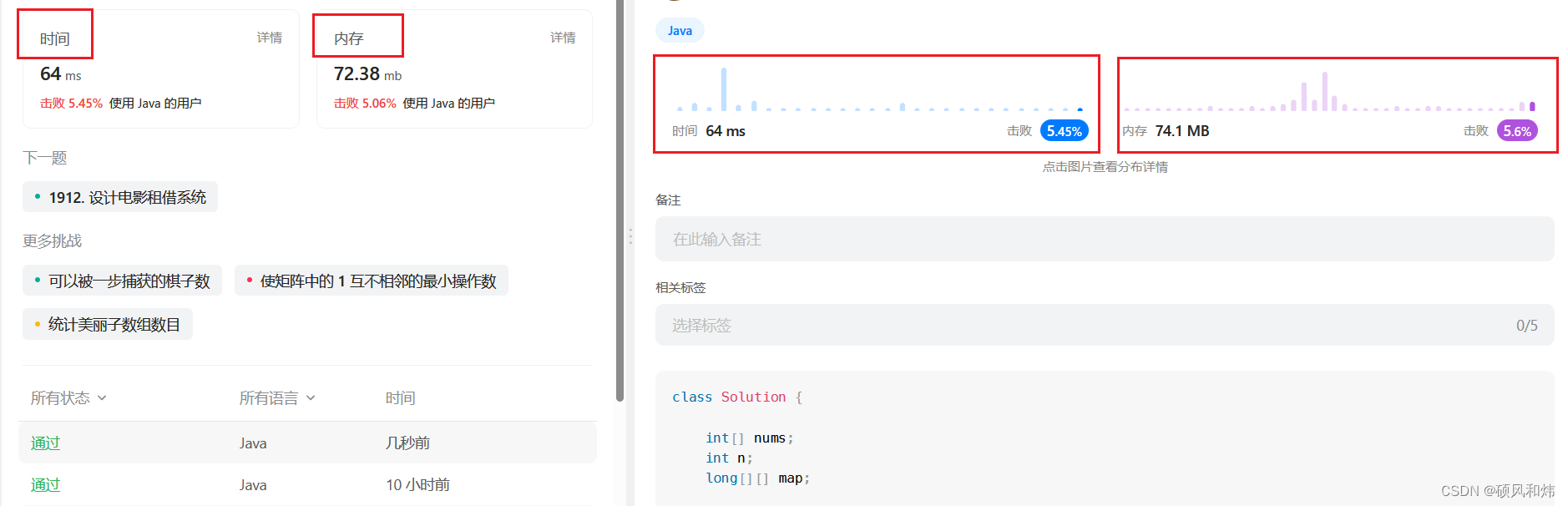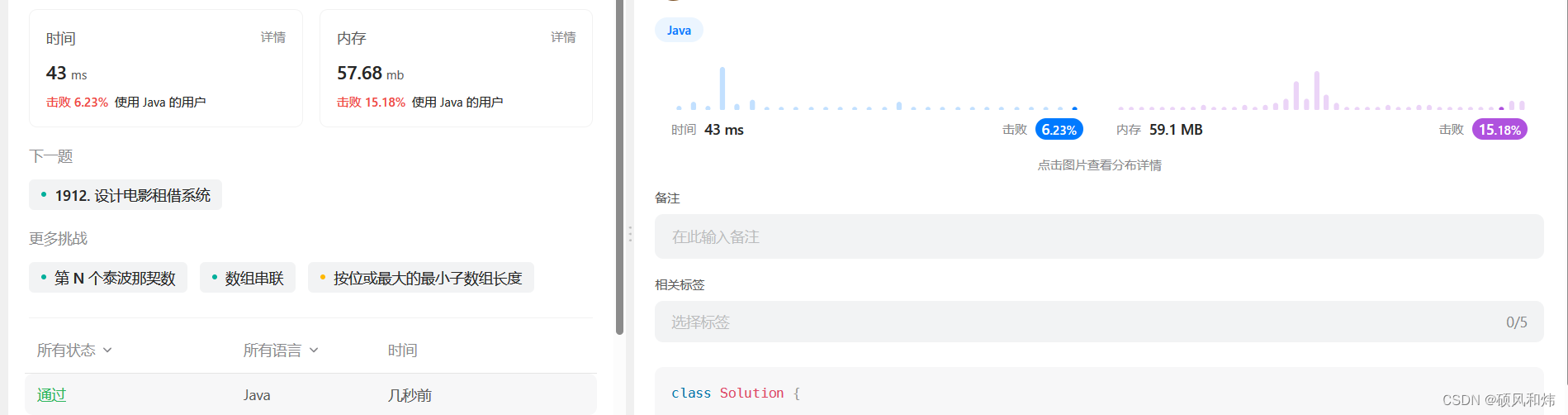##MSIPO技术圈 首页 IT技术 查看内容

# 【LeetCode: 1911. 最大子序列交替和 | 暴力递归=＞记忆化搜索=＞动态规划 】

2023-07-13🚀 算法题 🚀

🌲 算法刷题专栏 | 面试必备算法 | 面试高频算法 🍀
🌲 越难的东西,越要努力坚持，因为它具有很高的价值，算法就是这样✨
🌲 作者简介：硕风和炜，CSDN-Java领域优质创作者🏆，保研|国家奖学金|高中学习JAVA|大学完善JAVA开发技术栈|面试刷题|面经八股文|经验分享|好用的网站工具分享💎💎💎
🌲 恭喜你发现一枚宝藏博主,赶快收入囊中吧🌻
🌲 人生如棋，我愿为卒，行动虽慢，可谁曾见我后退一步？🎯🎯

 🚀 算法题 🚀## ⛲ 题目描述

1 <= nums.length <= 105
1 <= nums[i] <= 105

## 🌟 求解思路&实现代码&运行结果

### ⚡ 暴力法

#### 🥦 求解思路

1. 简单概括题目的意思:这是一道子序列类型的题目，每个位置可以选择，也可以不选，让我们求得nums数组中任意子序列的最大交替和。(最大交替和的定义请看题目)
2. 具体怎么求解呢？我们对题目进行一个拆分，发现最终求解的问题规模是可以转换为更小的问题规模进行求解的，所以我们通过递归的思路来求解。
3. 问题又来了？怎么设计递归函数呢？这个就需要大家认真考虑了。
4. 我们假设定义递归函数process(index,flag)，函数的含义是从index位置开始，flag=0代表此时已经选择了偶数个数，flag=1则相反，最终返回最大交替和。
5. 具体的状态是怎么转移的呢？我们可以分为以下俩种情况：如果当前选择了偶数个数，那么此时有俩种可能，可以之前并没有选择；也有可能是之前选择了奇数个数并且选择当前的数，俩者去较大值即可；同理可以推出如果选择了奇数个数的情况，最终再取得俩种情况的最大值即可。
6. 有了基本的思路，接下来我们就来通过代码来实现一下。

#### 🥦 实现代码

``````class Solution {

int[] nums;
int n;

public long maxAlternatingSum(int[] nums) {
n=nums.length;
this.nums=nums;
return process(0,0);
}

public long process(int index,int cnt){
if(index>=n) return 0;
long p1=0,p2=0;
if(cnt==0){
p1=Math.max(process(index+1,0),process(index+1,1)+nums[index]);
}else{
p2=Math.max(process(index+1,1),process(index+1,0)-nums[index]);
}
return Math.max(p1,p2);
}
}
``````

#### 🥦 运行结果### ⚡ 记忆化搜索

#### 🥦 求解思路

1. 因为在递归的过程中，会重复的出现一些多次计算的结果，我们通过开辟一个数组，将结果提前缓存下来，算过的直接返回，避免重复计算，通过空间来去换我们的时间。

#### 🥦 实现代码

``````class Solution {

int[] nums;
int n;
long[][] map;

public long maxAlternatingSum(int[] nums) {
n=nums.length;
this.nums=nums;
map=new long[n+1];
for(int i=0;i<=n;i++){
for(int j=0;j<2;j++){
map[i][j]=-1;
}
}
return process(0,0);
}

public long process(int index,int cnt){
if(index>=n) return 0;
if(map[index][cnt]!=-1) return map[index][cnt];
long p1=0,p2=0;
if(cnt==0){
p1=Math.max(process(index+1,0),process(index+1,1)+nums[index]);
}else{
p2=Math.max(process(index+1,1),process(index+1,0)-nums[index]);
}
map[index][cnt]=Math.max(p1,p2);
return map[index][cnt];
}
}
``````

#### 🥦 运行结果### ⚡ 动态规划

#### 🥦 求解思路

1. 有了递归，有了记忆化搜索，接下来就是动态规划了，直接上手。

#### 🥦 实现代码

``````class Solution {

int[] nums;
int n;
long[][] dp;

public long maxAlternatingSum(int[] nums) {
this.n=nums.length;
this.nums=nums;
dp=new long[n+1];
dp[n]=dp[n]=0;
for(int index=n-1;index>=0;index--){
dp[index]=Math.max(dp[index+1],dp[index+1]+nums[index]);
dp[index]=Math.max(dp[index+1],dp[index+1]-nums[index]);
}
return dp;
}
}
``````

#### 🥦 运行结果## 💬 共勉

 最后，我想和大家分享一句一直激励我的座右铭，希望可以与大家共勉！